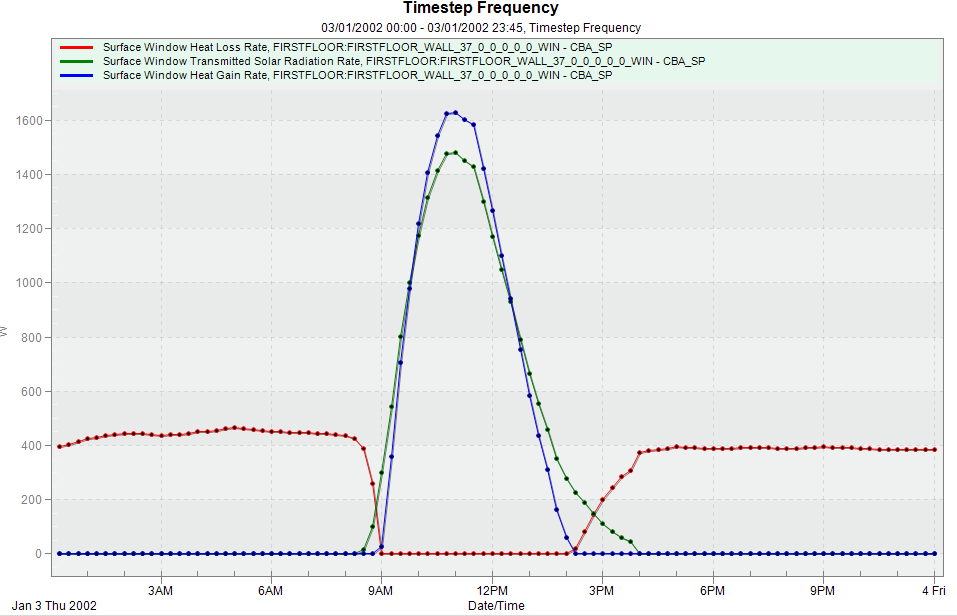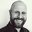Question-and-Answer Resource for the Building Energy Modeling Community
Get started with the Help page

# E+ outputs for building heat losses

Dear all,

I am trying to find out the correct variables of defining the heat loss of one building. What I am looking for are:Heat loss from the wall, roof, window, floor and infiltration/ventilation.

Up to now, after doing the searching and going through the InputOupt EP reference, what I come up with is:

Heat losses from walls, roof, ground floor = Surface Inside Face Conduction Heat Transfer Rate [W]

Heat losses from windows = Surface Window Heat Loss Rate [W]

Heat losses from infiltration = Zone infiltration Sensible Heat Loss

Heat losses from ventilation = Zone Ventilation Sensible Heat Loss

However, when I am looking at the results that the surface window heat loss rate is zero value for some time period(around 10am-3pm). I am wondering if I am looking at the right output.

one results shown below which is one window heat flow, including window heat gain, heat loss and transmitted solar radiation.

From the definition:

Surface Window Heat Gain Rate: The total heat flow to the zone from the glazing, frame and divider of an exterior window when the total heat flow is positive.

Surface Window Heat Loss Rate : The absolute value of the total heat flow through an exterior window when the total heat flow is negative.

Window heat gain = Window Heat Gain [W] - Window Heat Loss [W] - Window Transmitted Solar [W]

When calculate this window heat gain, I got positive numbers roughly around 10am-3pm. However, from the basic calculation of heat loss = UA(Tin-Tout), (the indoor temp is 18C, outdoor temp is around 2C), for this case, there should be always loss from window, not gains.Thanks

edit retag close merge delete

Sort by » oldest newest most votedThe period where the heat loss is zero is when the heat gain is non-zero and vice versa. I think you'll need to use both of those variables to get to a balance of energy flows.

more

Thanks Jamie, you are right, if looking at the heat flows which should be = Surface Window Heat Gain - Surface Window Heat Loss - transmitted solar radation. For example if we looking at the results, the heat flow is positive. However, what I am trying to find out is the heat loss through the window, normally can be calculated by the equation: UA(Tin-Tout). When the outdoor temp is lower than indoor, there should be losses from the window. I am trying to find out this results from E+.

Hey Jamie, I've update my questions more detail, if you could have a look and let me know your thoughts on it. thanks

Yes, looks like you have a point. I'm not sure how to explain that but it does look like there must be something missing there.